# Properties of Shapes: Rectangles, Squares and Rhombuses

Lesson Transcript
Instructor: Jeff Calareso

Jeff teaches high school English, math and other subjects. He has a master's degree in writing and literature.

Different objects come in different shapes that help achieve their purpose. Learn about the properties of different shapes, including rectangles, squares, and rhombuses. Updated: 08/26/2021

## Studying Shapes

Let's talk about shapes. There are all kinds of shapes, and they serve all kinds of purposes. If the wheels on your bike were triangles instead of circles, it would be really hard to pedal anywhere. And, if bowling balls were cubes instead of spheres, the game would be very different.

Here, we're going to focus on a few very important shapes: rectangles, squares and rhombuses. All of them are quadrilaterals. That just means they all have four sides. Remember that 'quad' means 'four.' That means no pentagons or octagons will be discussed here. Sorry, fans of Department of Defense headquarters or, um, stop signs. But, even though they all have four sides, they all have their own special properties that make them unique.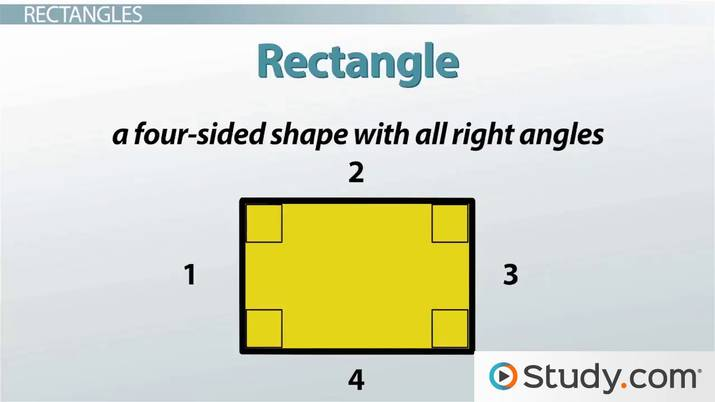An error occurred trying to load this video.

Try refreshing the page, or contact customer support.

Coming up next: Properties of Shapes: Triangles

### You're on a roll. Keep up the good work!

Replay
Your next lesson will play in 10 seconds
• 0:05 Studying Shapes
• 0:52 Rectangles
• 2:03 Squares
• 3:24 Rhombuses
• 4:58 Lesson Summary
Save Save

Want to watch this again later?

Timeline
Autoplay
Autoplay
Speed Speed

## Rectangles

Let's start with rectangles. A rectangle is a four-sided shape with all right angles. If you want to know if a shape is a rectangle, you just have two tests. Is it four-sided? And, are all the angles 90 degrees? If both answers are yes, then you're looking at a rectangle.

Rectangles are everywhere. Think about your average room. What's a rectangle in it? Doors, tables, windows, posters on the walls - they're all four-sided shapes with all right angles. Even the screen you're looking at right now is probably a rectangle.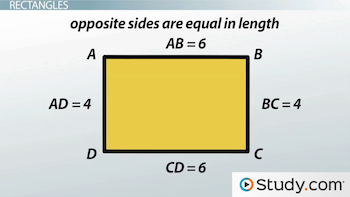Rectangles have a few special properties. First, opposite sides are parallel. Second, opposite sides are equal in length. In the rectangle above, we know side AB is parallel to side CD, and BC is parallel to AD. Also, if we know AB is 6, then so is CD. If BC is 4, then so is AD.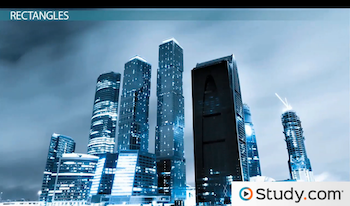The fun thing about rectangles is that each pair of opposite sides can be a totally different length than the other pair. You can have a super skinny rectangle like the skyscraper above or a very even one like the old album cover below.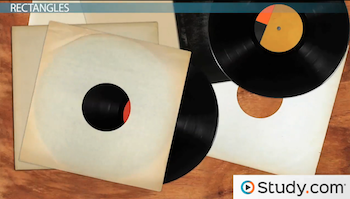## Squares

That old album cover fits both the definition of a rectangle and the definition of our next shape, the square. Squares are a very special subset of rectangles. A square is a four-sided shape with all right angles and sides of equal length.

Does that definition look familiar? Here are the steps to define a square: Is it four-sided? Are all the angles 90 degrees? If yes, then you have a rectangle. If all the sides are the same length, then it's not only a rectangle, it's also a square. That means that all squares are rectangles. But not all rectangles are squares, since a rectangle's pairs of sides can have different lengths.

Just like rectangles, squares are everywhere. In addition to the album cover, think about the spaces on a chessboard, stamps, floor tiles and even a snack of crackers and cheese. Because each side of a square has the same length, you don't need to be given much information to solve most problems.

For example, if you see the square below where you know one side is 5, then you know all the other sides are 5 as well. So, the perimeter of this square is 5 * 4, or 20. The area of a square is s^2, or one side squared. So, the area of this square is 5^2, which is 25.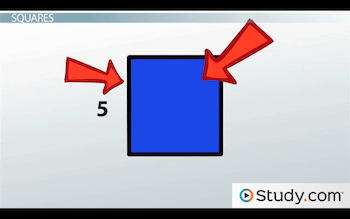To unlock this lesson you must be a Study.com Member.

### Register to view this lesson

Are you a student or a teacher?